# Prime numbers - 5th grade (10y) - math problems

1. The smallest numberWhat is the smallest number that can be divided by both 5 and 7
2. Dinosaurs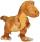More than 30 and less than 60 dinosaurs have met at the pond. A quarter of them bathed and 1/7 saws and the rest gripped. How many were at the pond? How many were there?
3. Czech crownsOldrich has one crown. Peter has five crowns coin, a two crown coin and a one-crown coin. Radek has twenty-crown banknote, ten banknote and five-crown coin. The boys got one fifty-one crown and one crown coin. How can they share the money fairly when they
4. LCMCommon multiple of three numbers is 3276. One number is in this number 63 times, second 7 times, third 9 times. What are the numbers?
5. Buses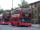At the bus stop is at 10 o'clock met buses No. 2 and No. 9. Bus number 2 runs at an interval of 4 minutes and the bus number 9 at intervals of 9 minutes. How many times the bus meet to 18:00 local time?
6. Lesson exercising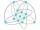The lesson of physical education, pupils are first divided into three groups so that each has the same number. The they redistributed, but into six groups. And again, it was the same number of children in each group. Finally they divided into nine equal g
7. Birthdate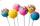Jane on birthday brought 30 lollipops and 24 chewing gum for their friends. How many friends has, if everyone receives the same number of lollipops and chewing gums? How much chewing gum and lollipops got any friend?
8. FractionsSort fractions ? by its size. Result write as three serial numbers 1,2,3.
9. CherriesCherries in the bowl can be divided equally among 19 or 13 or 28 children. How many is the minimum cherries in the bowl?
10. Class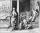When Pythagoras asked how many students attend the school, said: "Half of the students studying mathematics, 1/4 music, seventh silent and there are three girls at school." How many students had Pythagoras at school?
11. Primes 2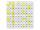Which prime numbers is number 2025 divisible?

We apologize, but in this category are not a lot of examples.
Do you have an interesting mathematical word problem that you can't solve it? Submit math problem, and we can try to solve it.

We will send a solution to your e-mail address. Solved examples are also published here. Please enter the e-mail correctly and check whether you don't have a full mailbox.

Please do not submit problems from current active competitions such as Mathematical Olympiad, correspondence seminars etc...